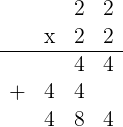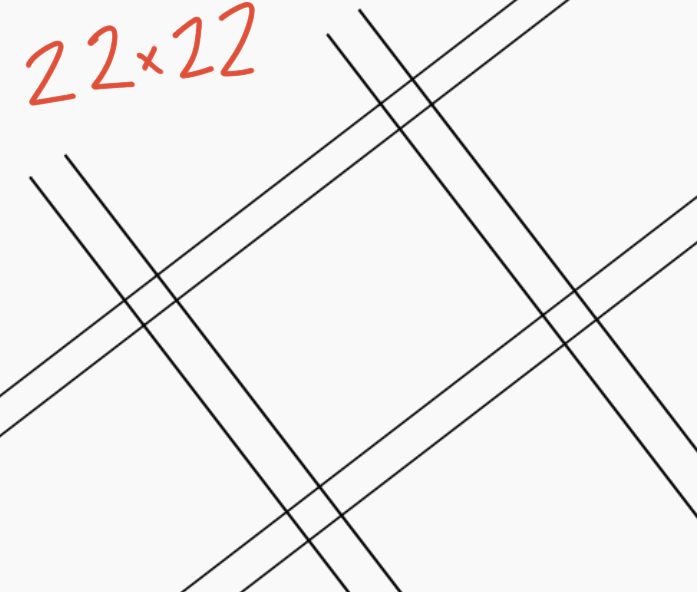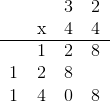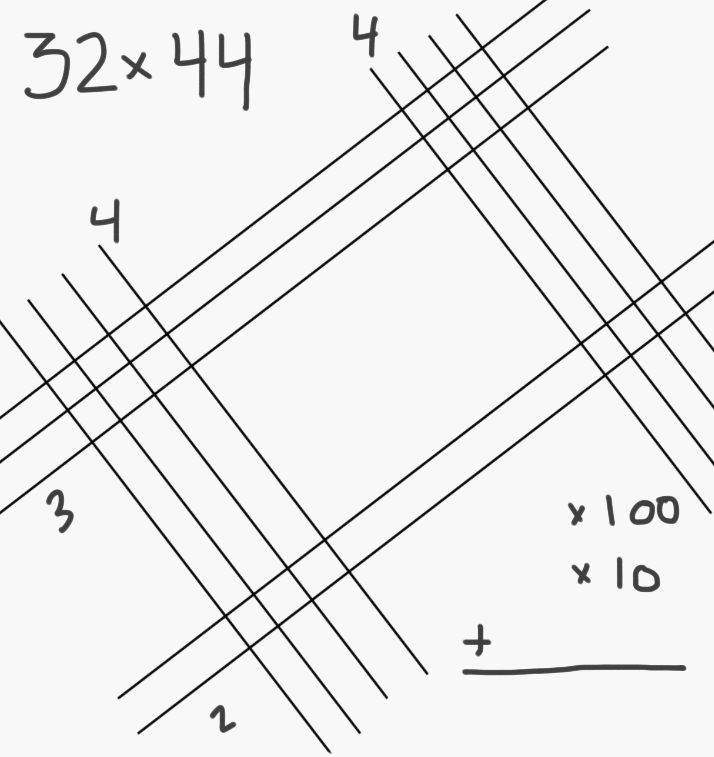# Visual Multiplication with Lines

Instructor: Nick Rogers
Multiplying large numbers can be thorny. You can multiply two digit numbers by simply drawing sets of lines and counting their intersections. This quick trick will help you visualize how multiplying works. Sometimes it's even faster!

## Multiplication Techniques

Since multiplication is built on addition, you can multiply numbers by using counting. This article will show you how to multiply using the visual multiplication with lines technique. We will examine a simple example, and then a more complicated example. As always, practice makes perfect, so get out some paper and follow along with the examples. Then try some of your own.

## Practice Problem: 22 x 22

Let's first try multiplying 22 x 22. We will use two techniques - classic algebraic multiplication, and then visual multiplication with lines.

### Traditional (Algebraic) Multiplication

Multiplication of large numbers works because the numbers themselves are made up of smaller numbers. Think of 22. We can write this as follows:

22 = 20 + 2.

Now if we want to multiply 22 by 22, we can think of splitting up the problem into two smaller problems.

22 x 22

= 22 x (20 + 2)

= 440 + 44

= 484

You may have seen this method before. We usually write it out this way:### Multiplication with Lines

Now, let's try to visualize what is happening using the method of lines. Draw one set of lines for each digit in the multiplication problem. In this example (22 x 22), we have four sets with two lines each.

The first set of lines that are moving up (left to right) represent the first digit of the first number (2). The second set of lines that are sloping upward represent the second digit of the first number (2). In the same way, the two sets of downward sloping lines represent the two digits of the second number.Once you have drawn the sets of lines, you can simply count intersections, and read off your answer. The intersection on the left tells us the hundreds, the two intersections in the middle tell us the number of tens, and the intersection to the right tells us the number of ones. Notice that the middle number counts both of the intersections in the middle of the picture.

We can write this process down using the hundreds', tens', and ones' places:

Left intersection (hundreds' place): 4 x 100 = 400

Middle intersections (tens' place): 8 x 10 = 80

Right intersection (ones' place): 4 x 1 = 4

400 + 80 + 4 = 484

Pretty neat, right? So is one method faster than the other one? Well in the example we looked at above, it may seem that the method of lines is faster. You can read off the answer pretty quickly and don't even have to do extra multiplication and addition. This is not always the case.

## Practice Problem: 32 x 44

### Traditional (Algebraic) Multiplication

We can split this problem up in the same way as the first one, and approach it as the sum of two simpler multiplications:

32 x 44

= 32 x (40 + 4)

= (32 x 40) + (32 x 4)

= 1280 + 128

= 1408

If you found it difficult to multiply 32 x 40 or 32 x 4, you can think of breaking these problems down even further by writing 32 as (30 + 2):

32 x 4

= (30 + 2) x 4

= (30 x 4) + (2 x 4)

= (120 + 8)

= 128

and since 40 = 10 x 4, then 32 x 40 is simply ten times the answer we got above, or 1280.

Again, you may have seen this calculation carried out as follows:Part of what makes this question more challenging is the 'carrying' over of digits. When we add 1280 and 128 together, we cannot simply 'add down the columns' since this results in a number greater than nine (in the third column from the left, 2 + 8 = 10). The 1 'carries' over and affects the calculation in the next column. Let's examine what happens in the visual method for this problem:

### Visual Multiplication with LinesWhy is this example more difficult? The answer lies in the tens' position. We cannot just combine 12 + 20 + 8 for an answer to get 12208! Let's unravel some of the math behind the scenes:

Left intersection (hundreds' place): 12 x 100 = 1200

Middle intersections (tens' place): 20 x 10 = 200

To unlock this lesson you must be a Study.com Member.
Create your account

### Register to view this lesson

Are you a student or a teacher?

### Unlock Your Education

#### See for yourself why 30 million people use Study.com

##### Become a Study.com member and start learning now.
Back
What teachers are saying about Study.com

### Earning College Credit

Did you know… We have over 200 college courses that prepare you to earn credit by exam that is accepted by over 1,500 colleges and universities. You can test out of the first two years of college and save thousands off your degree. Anyone can earn credit-by-exam regardless of age or education level.

To learn more, visit our Earning Credit Page

### Transferring credit to the school of your choice

Not sure what college you want to attend yet? Study.com has thousands of articles about every imaginable degree, area of study and career path that can help you find the school that's right for you.

Create an account to start this course today
Try it risk-free for 30 days!

Support